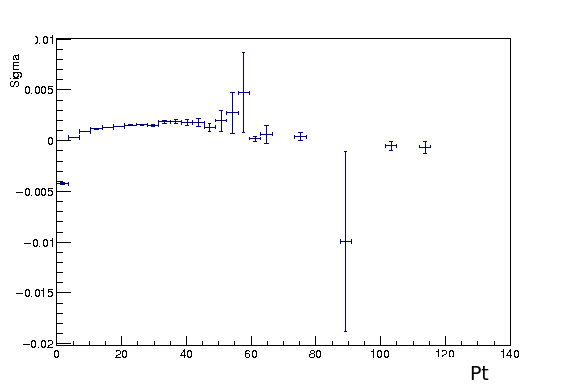# Calculate the percentage

Hi Everyone,

I made some histograms, and now I would like to calculate the percentage of how those histogram increase or decrease .

you can find bellow example of plot
Could someone explain me how to do it please,

Any help and ideas would be much appreciated!
CheersI’m afraid I have no idea what you want.

1 Like

I want determinate the percentage of how sigma are increase and decrease in the plot

``````for (int i = 1; i < Pt_Histo->GetNbinsX(); i++) {
std::cout << Pt_Histo->GetBinContent(i + 1) - Pt_Histo->GetBinContent(i) << std::endl;
}
``````
1 Like

Hi Coyote,